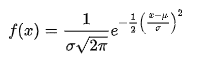# Arithmetic progression, Geometric progression and Harmonic progression

Summary:
It looks as if the AP, GP, HP can be used to build any function.
How do I build functions by using Arithmetic Sequence, Geometric Sequence, Harmonic Sequence?
Is it possible to create all the possible function by using these sequences?

Thanks!

mfb
Mentor
How do you "use a sequence to build a function"? What does that mean?

Sequences are numbers with common difference. f(x) is a sequence and g(x) is a sequence. If there is common difference then there is consistency among numbers. If there is consistency among numbers then I can build an equation.
Example:
The values in the second column of the below table have consistent numbers for given input 'x' for drawing a graph of f(x) or g(x) .
So the equation is:

y = f(x) = x + 2;

xf(x) = y
-20
-11
02
13
24

y = g(x) = 3x + 2

xg(x) = y
-3-7
-2-4
-1-1
02
15
28
311

So, all functions have consistent numbers?Last edited:
mfb
Mentor
Not all functions are one of these progressions. First of all, these progressions are only defined for integers, so you'll never catch functions defined on other sets, like the real numbers. But it doesn't even work for integers. Simple counterexamples are f(x)=x3 or g(x)=|x| or h(x)=sin(x). The Gaussian distribution you posted is a counterexample, too. Only a very limited set of functions can be made with the three progressions you listed.

Only a very limited set of functions can be made with the three progressions you listed.
If there is a pattern of numbers that have consistent numbers in it then any function can be created. No?

Last edited:
pbuk